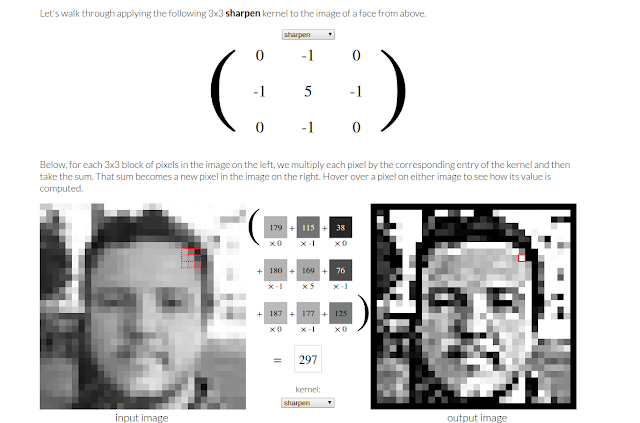Sunday, December 10, 2017

Week 1 : fast.ai - 1 v2 - CNNs, Kernels, Optimal Learning Rate

Deep Learning is essentially a particular way of doing Machine Learning where you give your system a bunch of examples and then it learns the rules and representations vs manually programming the rules

We have interesting applications today like Cancer diagnosis, Language Translation, Inbox by Google, Style Transfer, Data Center Costs optimization, Playing the Game Of Go among others that are powered by Deep Learning. Jeremy also emphasizes all the negatives that come with the growth of Deep learning like algorithmic bias, societal implications, job automation, etc

With Deep Learning we need an infinitely flexible mathematical function that can solve any problem. Such a function would be quite large with lots of parameters and we have to fit those parameters in a fast and scalable way using GPUs.

The fast.ai philosophy is closely modeled after some of the concerns Paul Lockhart voiced in his essay A Mathematician's Lament which pushes you to start by doing right away and then gradually peel back the layers, modify and look under the hood. The general feeling out there is that there is a survival bias problem in the Deep Learning space which is typified by this Hacker News post. The only currency that should matter is how well you're able to use these tools to solve problems and generate value.

Convolutions

CNNs are the most important architecture for Deep Neural Networks. They're the state of the art for solving problems in many areas of Image Processing, NLP, Speech Processing, etc

The basic structure of a CNN is the convolution which on its own is a relatively straightforward process. A convolution is a linear operation that finds interesting features in an image. Performing one instance of the convolution operation (element-wise multiplication and addition) requires the following steps

• Identify kernel matrix - this is typically a 3 x 3 matrix or in some cases a 1 x 1 matrix
• Pass kernel matrix over image (see figure below)
• Perform element-wise multiplication between kernel and overlaying image pixels (see red box in image below)
• Sum all the elements in the resulting matrix ( in the figure below, the sum is 297)
• Assign the sum as the new pixel value for the center pixel in the overlayed image crop in the activation map
• This operation is repeated until you've completed passes over the entire image

There are other parameters like kernel stride and padding that determine the dimension of the activation maps. I'll be doing a more in-depth post on Convolutional Neural Network to discuss theses and the full CNN pipeline.Courtesy of setosa.io/ev/image-kernels/
In the figure above, we used the sharpen kernel. There are also a few other predefined kernels like sobel, emboss, etc. In a typical CNN pipeline, we start with randomly initialized convolution filters, apply a non-linear ReLU activation (remove negatives) and then use SGD + backpropagation to find the best convolution filters. If we do this with enough filters and data we end up with a state of the art image recognizer. CNNs are able to learn filters that detect edges and other simple image characteristics in the lower layers and then use those to detect more complex image features and objects in the deeper layers.

To train an image classifier using the fast.ai library you need to
• Select a starting architecture: resnet34 is a good option to start with.
• Determine the number of epochs: start with 1, observe results and then run multiple epochs if needed
• Determine the learning rate: Using the strategy in the Cyclical Learning Rate paper, we keep increasing learning rate until the loss stars decreasing. This will probably take less than one epoch if you have a small batch size. From the figure below, we want to pick the largest learning rate as long as the loss is still decreasing  (in this case learning_rate = 0.01)
• Train learn objectCourtesy of fast.ai
Highlights
• We used a pre-trained ResNet34 to train a CNN on data from the Cats vs Dogs Kaggle competition and obtained > 99% accuracy
• Used a new method (Cyclical Learning Rates for Training Neural Networks) to determine the optimal learning rate which determines how quickly we update our weights.